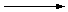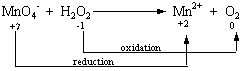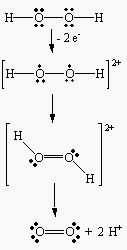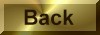Practice Problem 5

An endless number of balanced equations can be written for the reaction between the permanganate ion and hydrogen peroxide in acidic solution to form the manganese (II) ion and oxygen:

 MnO4-(aq) + H2O2(aq)Mn2+(aq) + O2(g)

Use the half-reaction method to determine the correct stoichiometry for this reaction.

Solution

STEP 1: Write a skeleton equation for the reaction.

MnO4- + H2O2Mn2+ + O2

STEP 2: Assign oxidation numbers to atoms on both sides of the equation.
 MnO4- + H2O2Mn2+ + O2 +7 -2 +1-1 +2 0

STEP 3: Determine which atoms are oxidized and which are reduced.STEP 4: Divide the reaction into oxidation and reduction half-reactions and balance these half-reactions. We balanced the reduction half-reaction in the previous exercise.

 Reduction: MnO4- + 8 H+ + 5 e-Mn2+ + 4 H2O

To balance the oxidation half-reaction, we have to remove two electrons from a pair of oxygen atoms in the -1 oxidation state to form a neutral O2 molecule.

 Oxidation: H2O2O2 + 2 e-

We can then add a pair of H+ ions to the products to balance both charge and mass in this half-reaction

 Oxidation: H2O2O2 + 2 H+ + 2 e-

as shown in the following figure.STEP 5: Combine the two half-reactions so that electrons are neither created nor destroyed. Two electrons are given off during oxidation and five electrons are consumed during reduction. We can combine these half-reactions so that electrons are conserved by using the lowest common multiple of 5 and 2.

 2(MnO4- + 8 H+ + 5 e-Mn2+ + 4 H2O) + 5(H2O2O2 + 2 H+ + 2 e-) ________________________________________________________ 2 MnO4- + 5 H2O2 + 16 H+2 Mn2+ + 5 O2 + 10 H+ + 8 H2O

STEP 6: Balance the remainder of the equation by inspection, if necessary. The simplest balanced equation for this reaction is obtained when 10 H+ ions are subtracted from each side of the equation derived in the previous step.

2 MnO4-(aq) + 5 H2O2(aq) + 6 H+(aq)2 Mn2+(aq) + 5 O2(g) + 8 H2O(l)